# “standardized” version of the maximum

For the first homework, there was a tricky question in problem 29, chapter 5. Here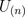is the maximum of n random variables i.i.d. uniformly distributed on the unit interval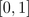. I gave a hint last week about the cumulative distribution function for the maximum, i.e.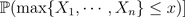is equal to the probability that all are smaller than,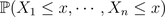Then, we use independent to obtain that this probability is a product, of equal quantities since all random variables are identically distributed, i.e.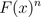Then, the exercise ask the followingi.e. find a standardized version of that the maximum so that the cumulated distribution of that standardized version has a (non degenerated) limiting value. A hint is given in the answers, at the end of the book,Actually, the question is not that simple (see here for the history of that question).
What I said during the course is that if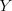is a random variable with finite variance, thenis a standardized (or normalized) version of, in the sense that it is centered, i.e.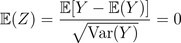and with a unit variance, i.e.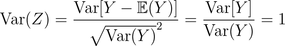This is the kind of standardization (or normalization) that is used in the central limit theorem i.e. it is interesting when we study the core of our distribution (i.e. the mean).
Here we focus on the maxima (not on the expected value). Note that here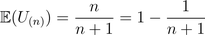while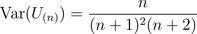(up to some typing mistakes). Thus, our previous standardization would be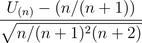that can be simplified as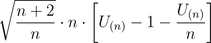Hence, that random variable can be approximated by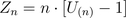since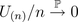as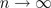. Here, it is then possible to get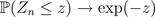since if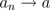, then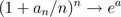(see the prof of the central limit theorem we got a few days ago).
But this is usually not the way we work with maxima. Actually, Fréchet, Fisher, Tippett, Gnedenko proved that the appropriate standardization to work with maxima is to consider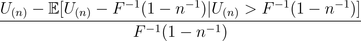where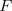is the cumulative distribution of the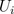‘s (the random variables used to build up the maximum). This work since the‘s have a finite support, i.e. theare bounded, with an upper limit (here 1).
Note that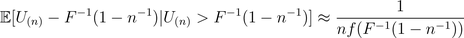assuming that the density associated withexists. Hence, here the standardization becomes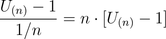which is exactly the one that John Rice is suggesting… And the proper motivation comes from extreme value theory, but it is a bit far away from what we shall see in that course…

This site uses Akismet to reduce spam. Learn how your comment data is processed.